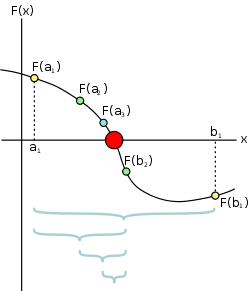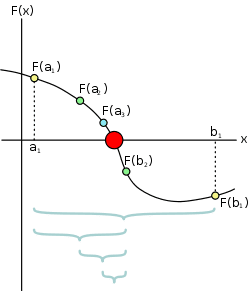# ROOT / C++ Questions

• Comp Sci
Gold Member
I have three problems that I've been trying to tackle for a while now.

1) I have a code for the bisection method. I would like to use TLine *line = new TLine function to create the lines that you normally see in the bisection method.These lines from a to F(a) are the ones I am talking about.

I have a for statement that does the following:

Code:
for(int i = 0; i < 10; i++)  //try 10 steps
{
c = 0.5*(x1+x2);  // new midpoint
tempy= f->Eval(c);

w[ i ]=x1;
y[ i ]=x2;

if( fabs(tempy) < 0.00001 ) break;// found it!

if( tempy*f(x1)  > 0 ) x1 =c;
else x2=c;

cout<<"\nThe value is: ";
cout<< c <<"\n\n The function value at this point is: "<<tempy<<endl;

cout<<"x1 = "<<w[ i ]<<", x2 = " <<y[ i ]<<endl ;
cout<<"\n\n Function evaluated at x1 = "<<f->Eval(w[ i ])<<"\n\n Function evaluated at x2 = "<<f->Eval(y[ i ])<<endl;

}

And this works great! I get the x1 and x2 values that I need into the w[ i ]and y arrays, and I can even evaluate them at my function.

But when I type something like:

Code:
  TLine *line2 = new TLine(w[ i ], 0, w[ i ], f->Eval(w[ i ]));
TLine *line3 = new TLine(y[ i ], 0, y[i], f->Eval(y[ i ]));
line2->SetLineColor(kBlack);
line3->SetLineColor(kBlack);
line2->Draw("SAME");
line3->Draw("SAME");

In a different for statement, it ruins the code.
I'm guessing this is because when I create the TLine i am creating just one TLine called 'line2' and trying to feed it many different lines.
But, I do not know how to make it so that I can get TLine *linei where i goes through the for loop i++.

2) The exponential function.
I am using root and I want to graph a function that looks like the following:
v = (a/b) (1-e^(-bt))

I try to define the exponential function part as float expo = exp(-b*t)
but this doesn't seem to work. I am guessing the exponential function does not work with root.
How can I get this to work? I know that there is some TMath:: things out there, but I have never been able to get them to work.

3. I am trying to write text on my graph, but It wont let me use a variable.

I have a simple TF1 graph that depicts free fall motion. I have solved it using the quadratic formula to find the root aka the time it takes for the particle to reach the floor.
I have this root labeled as t.

In my code I have the following:

Code:
TF1 *fa1 = new TF1("fa1","*x*x+*x+",0,2*t);
fa1->SetParameters(-0.5*g,v,h);
fa1->SetTitle("Projectile Motion");
fa1->GetYaxis()->SetTitle("Height in meters");
fa1->GetXaxis()->SetTitle("Time in seconds");
TLine *line = new TLine(0,0,2*t,0);
line->SetLineColor(kBlue);
TText *z = new TText(.5, -20, "Solution for t = ", t);
z->SetTextSize(0.02);
fa1->Draw();
line->Draw();
z->Draw();

As you can see, I am trying to use TText to let me write on the graph the "solution for t = " t.
But this doesn't work. The TText doesn't seem to know what to do when I simply write t.

Any suggestions on these three problems?

I thank you all greatly.

Last edited by a moderator:

Mark44
Mentor
You have an array index that is causing the following text to be in italics, and will "eat" some of your code. I'm going to lock your post temporarily and fix things, and will then reopen it.

•RJLiberator
Mark44
Mentor
OK, it's back open again. If you use i as the index on an array, the sequence of characters [i] gets mistakenly taken as BBCode for italics, so x[i] turns into just x, with the characters following showing up in italics. Aside from the weird change to italics, you also lose information about the array index.

•RJLiberator
Gold Member
Damn. Thanks for checking in on that for me.

This is a tough assignment, already stayed up until 4-5am last night working on it. Have some kinks as mentioned in this post to work on tonight, will likely be up at it again all night.

I don't expect anyone here to know thoroughly about ROOT but perhaps some of the C++ in the code someone may be able to help me out with.

TLine *line2 = new TLine(w[ i ], 0, w[ i ], f->Eval(w[ i ]));

How does it break the code in the other function and are you certain this snippet is the only bit that is causing it to fail?

Mark44
Mentor
I have three problems that I've been trying to tackle for a while now.

1) I have a code for the bisection method. I would like to use TLine *line = new TLine function to create the lines that you normally see in the bisection method.These lines from a to F(a) are the ones I am talking about.

I have a for statement that does the following:

Code:
for(int i = 0; i < 10; i++)  //try 10 steps
{
c = 0.5*(x1+x2);  // new midpoint
tempy= f->Eval(c);

w[ i ]=x1;
y[ i ]=x2;

if( fabs(tempy) < 0.00001 ) break;// found it!

if( tempy*f(x1)  > 0 ) x1 =c;
else x2=c;

cout<<"\nThe value is: ";
cout<< c <<"\n\n The function value at this point is: "<<tempy<<endl;

cout<<"x1 = "<<w[ i ]<<", x2 = " <<y[ i ]<<endl ;
cout<<"\n\n Function evaluated at x1 = "<<f->Eval(w[ i ])<<"\n\n Function evaluated at x2 = "<<f->Eval(y[ i ])<<endl;

}

And this works great! I get the x1 and x2 values that I need into the w[ i ]and y arrays, and I can even evaluate them at my function.

But when I type something like:

Code:
  TLine *line2 = new TLine(w[ i ], 0, w[ i ], f->Eval(w[ i ]));
TLine *line3 = new TLine(y[ i ], 0, y[i], f->Eval(y[ i ]));
line2->SetLineColor(kBlack);
line3->SetLineColor(kBlack);
line2->Draw("SAME");
line3->Draw("SAME");

In a different for statement, it ruins the code.
What do you mean, "it ruins the code"?
RJLiberator said:
I'm guessing this is because when I create the TLine i am creating just one TLine called 'line2' and trying to feed it many different lines.
But, I do not know how to make it so that I can get TLine *linei where i goes through the for loop i++.
line2 and line3 are pointers to TLine instances. If you need to save a bunch of these things you could create an array of pointers to type TLine.

Something like this, where n is a constant. Caveat emptor -- I haven't checked this code.
C:
TLine * lines[n] ;
lines = new TLine(w[ i ], 0, w[ i ], f->Eval(w[ i ]));
// etc. for the other array elements
RJLiberator said:
2) The exponential function.
I am using root and I want to graph a function that looks like the following:
v = (a/b) (1-e^(-bt))

I try to define the exponential function part as float expo = exp(-b*t)
but this doesn't seem to work. I am guessing the exponential function does not work with root.
How can I get this to work? I know that there is some TMath:: things out there, but I have never been able to get them to work.
I looked at some docs for TMath and ROOT (many of the links to docs at CERN seem to be broken). As far as I can tell, there is no exp() function, but there is a Power() function and a member named E. You have to include xmath.h.
To get ##e^{-bt}## you need to do something like this:
C:
v = a/b * (1 - Power((const double &)E, (const double &) (-b*t));

RJLiberator said:
3. I am trying to write text on my graph, but It wont let me use a variable.

I have a simple TF1 graph that depicts free fall motion. I have solved it using the quadratic formula to find the root aka the time it takes for the particle to reach the floor.
I have this root labeled as t.

In my code I have the following:

Code:
TF1 *fa1 = new TF1("fa1","*x*x+*x+",0,2*t);
fa1->SetParameters(-0.5*g,v,h);
fa1->SetTitle("Projectile Motion");
fa1->GetYaxis()->SetTitle("Height in meters");
fa1->GetXaxis()->SetTitle("Time in seconds");
TLine *line = new TLine(0,0,2*t,0);
line->SetLineColor(kBlue);
TText *z = new TText(.5, -20, "Solution for t = ", t);
z->SetTextSize(0.02);
fa1->Draw();
line->Draw();
z->Draw();

As you can see, I am trying to use TText to let me write on the graph the "solution for t = " t.
But this doesn't work. The TText doesn't seem to know what to do when I simply write t.
The TText() constructor expects three arguments, not four. If you want to have it print, say, Solution for t = 4, you need to create a string that has the 4 in it, and then pass the string as the third arg in the TText() ctor.
RJLiberator said:
Any suggestions on these three problems?

I thank you all greatly.

•RJLiberator
What do you mean, "it ruins the code"?
line2 and line3 are pointers to TLine instances. If you need to save a bunch of these things you could create an array of pointers to type TLine.

Something like this, where n is a constant. Caveat emptor -- I haven't checked this code.
C:
TLine * lines[n] ;
lines = new TLine(w[ i ], 0, w[ i ], f->Eval(w[ i ]));
// etc. for the other array elements
I looked at some docs for TMath and ROOT (many of the links to docs at CERN seem to be broken). As far as I can tell, there is no exp() function, but there is a Power() function and a member named E. You have to include xmath.h.
To get ##e^{-bt}## you need to do something like this:
C:
v = a/b * (1 - Power((const double &)E, (const double &) (-b*t));

The TText() constructor expects three arguments, not four. If you want to have it print, say, Solution for t = 4, you need to create a string that has the 4 in it, and then pass the string as the third arg in the TText() ctor.

https://root.cern.ch/doc/master/TMath_8h_source.html#l00495

inline Double_t TMath::Exp(Double_t x)
{return exp(x); }

OP said they are trying to define exp() as a float, where it is expecting a Double_t?

Nice catch on TText() :-)

•RJLiberator
mfb
Mentor
I try to define the exponential function part as float expo = exp(-b*t)
That would give a single value. TF1 with the full function should be the right thing here. Note the ugly ROOT syntax which requires you to write it in quotation marks.
OP said they are trying to define exp() as a float, where it is expecting a Double_t?
Should be fine. Nearly all things in ROOT work with float, double, Double_t and so on in the same way. TTrees are the only counterexample I know of.

•RJLiberator
Gold Member
Hey guys, just wanted to thank you all for the help.

I haven't quite got the all the kinks fixed, but I did fix some of them.

The exponential function can be read as exp([parameter]*x] when I use TF1.

The lines for the bisection method could be fixed in a much simpler way then I had initially thought.